oror

## 如何在Excel中添加业务/工作日或小时数到日期？

### 在Excel中轻松添加日期/小时数/分钟数：

Kutools for Excel's 添加日期/时间/分钟 公式可以帮助您在Excel中快速将日期，小时或分钟数添加到日期中。 看截图：

Kutools for Excel 包含了比300更方便的Excel工具。 免费试用60天无限制。 立即下载免费试用版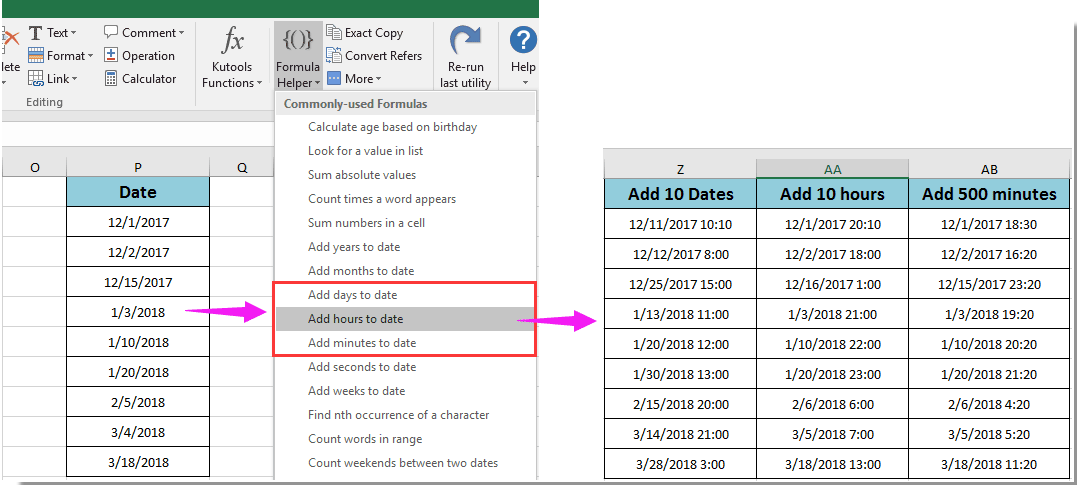Office选项卡在Office中启用选项卡式编辑和浏览，使您的工作更轻松......
Kutools for Excel - 最佳办公生产力工具将解决您的大部分Excel问题
• 重用任何东西： 将最常用或最复杂的公式，图表和其他任何内容添加到您的收藏夹中，并在将来快速重复使用它们。
• 超过20文本功能： 从文本字符串中提取数字; 提取或删除部分文本; 将数字和货币转换为英语单词...
• 合并工具：多个工作簿和表格合二为一; 合并多个单元格/行/列而不丢失数据; 合并重复行和总和...
• 拆分工具：根据价值将数据拆分为多个表格; 一个工作簿到多个Excel，PDF或CSV文件; 一列到多列......
• 粘贴跳过 隐藏/过滤行; 数和总和 按背景颜色; 创建邮件列表和 通过Cell的价值发送电子邮件...
• 超级过滤器： 创建高级过滤方案并应用于任何工作表; 排序 按周，日，频率等; 筛选 通过大胆，公式，评论......
• 超过300强大的功能; 适用于Office 2007-2019和365; 支持所有语言; 在公司轻松部署; 全功能60天免费试用。

#### 将公式日添加到具有公式的日期中###### 节省50％的时间，每天为您减少数千次鼠标点击！

1。 选择一个空白单元格，输入公式 = WORKDAY（A2,12）配方栏，然后按 输入 键。 看截图：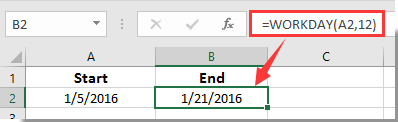1。 在公式中，A2是单元格包含要添加工作日的日期，12是您将添加到日期的工作日数。 请根据需要更改它们。

2。 用上面的公式，你会得到结果，不包括周末。 但计算后可能会包含一些假期。 如果您想排除周末和节假日，请使用此公式 = WORKDAY（A2，B2，C2）.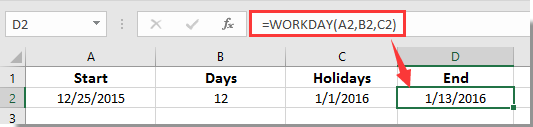3。 应用公式后，如果您得到一个5位数字，请将单元格格式转换为日期格式。

#### 将公司的营业时间添加到具有公式的日期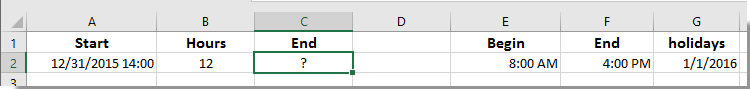1。 选择一个空白单元格（称为单元格C2），将以下公式输入到公式栏中，然后按 输入 键。

 =WORKDAY(A2,INT(B2/8)+IF(TIME(HOUR(A2),MINUTE(A2),SECOND(A2))+TIME(MOD(B2,8),MOD(MOD(B2,8),1)*60,0)>\$F\$2,1,0),\$G\$2:\$G\$2)+IF(TIME(HOUR(A2),MINUTE(A2),SECOND(A2))+TIME(MOD(B2,8),MOD(MOD(B2,8),1)*60,0)>\$F\$2,\$E\$2+TIME(HOUR(A2),MINUTE(A2),SECOND(A2))+TIME(MOD(B2,8),MOD(MOD(B2,8),1)*60,0)-\$F\$2,TIME(HOUR(A2),MINUTE(A2),SECOND(A2))+ TIME（MOD（B2,8），MOD（MOD（B2,8），1）* 60,0））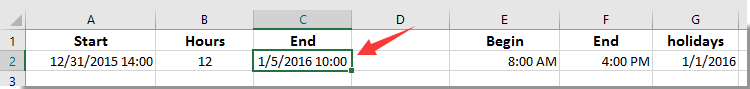1。 在公式中，A2是包含日期的单元格，B2包含您将添加的工作时间，E2和F2是工作时间的开始和结束时间，\$ G \$ 2是具体的假期日期。 你可以根据你的需要改变它们。

2。 如果您在应用此公式后得到一个数字，请将单元格格式更改为日期和时间格式。

#### Kutools for Excel - 最佳办公生产力工具提高80％的生产力

• 重用: 快速插入 复杂的公式，图表 以及你以前用过的任何东西; 加密单元格 密码; 创建邮件列表 并发送电子邮件...
• 超级方程式酒吧 （轻松编辑多行文字和公式）; 阅读布局 （轻松读取和编辑大量单元格）; 粘贴到过滤范围...
• 合并单元格/行/列 不丢失数据; 分裂细胞含量; 组合重复的行/列...防止重复的细胞; 比较范围...
• 选择复制或唯一 行; 选择空行 （所有细胞都是空的）; 超级查找和模糊查找 在许多工作簿中; 随机选择......
• 精确复制 多个单元格而不更改公式参考; 自动创建参考 多张表; 插入项目符号，复选框等等......
• 提取文本，添加文本，按位置删除， 删除空间; 创建和打印分页小计; 在单元格内容和注释之间转换...
• 超级过滤器 （将过滤方案保存并应用到其他工作表）; 高级排序 按月/周/日，频率等; 特殊过滤器 用粗体，斜体......
• 结合工作簿和工作表; 根据键列合并表; 将数据拆分为多个表格; 批量转换xls，xlsx和PDF...
• 超过300强大的功能。 支持Office / Excel 2007-2019和365。 支持所有语言。 在您的企业或组织中轻松部署。 全功能60天免费试用。#### Office选项卡为Office提供选项卡式界面，使您的工作更轻松

• 在Word，Excel，PowerPoint中启用选项卡式编辑和阅读，Publisher，Access，Visio和Project。
• 在同一窗口的新选项卡中打开并创建多个文档，而不是在新窗口中。
• 通过50％提高您的工作效率，每天为您减少数百次鼠标点击！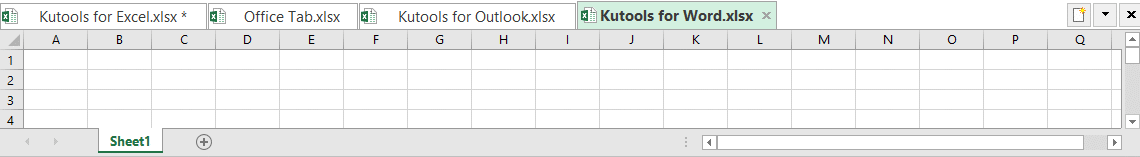Say something here...
symbols left.
###### or post as a guest, but your post won't be published automatically.
Loading comment... The comment will be refreshed after 00:00.
• To post as a guest, your comment is unpublished.
· 5 months ago
can some help me to get a formula for below condition
I am unable to get the time , If i do =WORKDAY.INTL(A2,B2,1) ---Output is : Deal Date: 9/11/2018 0:00, Where the time is not coming up, As time should come with 18:34 PM

A2: Deal Date :9/7/2018 18:34 PM
B2: Days: 2
Output is :New Deal Date: 9/11/2018 0:00

Required Output is 9/11/2018 18:34 PM - Plz help me with the formula!
--------------------
9/7/2018 - Friday , I need to add 2 days excluding weekends, So if i add 2 days to 9/7/2018(Friday ) its Tuesday (9/11/2018) by removing the Sat & Sun.
• To post as a guest, your comment is unpublished.
· 3 months ago
Hi Harish,
Sorry can't help you with that yet. Thanks for your comment.
• To post as a guest, your comment is unpublished.
· 5 months ago
How to add a cell in the excel says that maximum hours per day (i.e., 8 hours) The time calculation to be bound by 8 hours per day. Please help me if we can have the spread sheet cap the calculation at 8.?
• To post as a guest, your comment is unpublished.
· 6 months ago
How to get cells in excel formula (H6; H7; H8; H9)
Forma C2 = =WORKDAY(B2;MAX(ROUNDUP((E2-IF((COUNTIF(\$J\$4:\$J\$91;INT(B2))=0)*(WEEKDAY(B2;2)<=5);IF(MOD(B2;1)>\$H\$3;0;IF(MOD(B2;1)>=\$H\$5;(H3-MOD(B2;1))*24;(\$H\$3-MAX(MOD(B2;1);\$H\$2)-(\$H\$5-MAX(MOD(B2;1);\$H\$4)))*24));0))/((\$H\$3-\$H\$2-(\$H\$5-\$H\$4))*24);0);0);\$J\$4:\$J\$91)+\$H\$2+(IFERROR(CEILING(MOD((E2-IF((COUNTIF(\$J\$4:\$J\$91;INT(B2))=0)*(WEEKDAY(B2;2)<=5);IF(MOD(B2;1)>\$H\$3;0;IF(MOD(B2;1)>=\$H\$5;(\$H\$3-MOD(B2;1))*24;(\$H\$3-MAX(MOD(B2;1);\$H\$2)-(\$H\$5-MAX(MOD(B2;1);\$H\$4)))*24));0))/((\$H\$3-\$H\$2-(\$H\$5-\$H\$4))*24);1)-0,00000001;0,00000001);1)*(\$H\$3-\$H\$2-(\$H\$5-\$H\$4))*24+(IFERROR(CEILING(MOD((E2-IF((COUNTIF(\$J\$4:\$J\$91;INT(B2))=0)*(WEEKDAY(B2;2)<=5);IF(MOD(B2;1)>H3;0;IF(MOD(B2;1)>=\$H\$5;(\$H\$3-MOD(B2;1))*24;(\$H\$3-MAX(MOD(B2;1);\$H\$2)-(\$H\$5-MAX(MOD(B2;1);\$H\$4)))*24));0))/((\$H\$3-\$H\$2-(\$H\$5-\$H\$4))*24);1)-0,00000001;0,00000001);1)*(\$H\$3-\$H\$2-(\$H\$5-\$H\$4))*24>(\$H\$4-\$H\$2)*24)*(\$H\$5-\$H\$4)*24)/24

and C3 = =WORKDAY(B3;MAX(ROUNDUP((E3-IF((COUNTIF(\$J\$4:\$J\$91;INT(B3))=0)*(WEEKDAY(B3;2)<=5);IF(MOD(B3;1)>\$H\$3;0;IF(MOD(B3;1)>=\$H\$5;(H4-MOD(B3;1))*24;(\$H\$3-MAX(MOD(B3;1);\$H\$2)-(\$H\$5-MAX(MOD(B3;1);\$H\$4)))*24));0))/((\$H\$3-\$H\$2-(\$H\$5-\$H\$4))*24);0);0);\$J\$4:\$J\$91)+\$H\$2+(IFERROR(CEILING(MOD((E3-IF((COUNTIF(\$J\$4:\$J\$91;INT(B3))=0)*(WEEKDAY(B3;2)<=5);IF(MOD(B3;1)>\$H\$3;0;IF(MOD(B3;1)>=\$H\$5;(\$H\$3-MOD(B3;1))*24;(\$H\$3-MAX(MOD(B3;1);\$H\$2)-(\$H\$5-MAX(MOD(B3;1);\$H\$4)))*24));0))/((\$H\$3-\$H\$2-(\$H\$5-\$H\$4))*24);1)-0,00000001;0,00000001);1)*(\$H\$3-\$H\$2-(\$H\$5-\$H\$4))*24+(IFERROR(CEILING(MOD((E3-IF((COUNTIF(\$J\$4:\$J\$91;INT(B3))=0)*(WEEKDAY(B3;2)<=5);IF(MOD(B3;1)>H4;0;IF(MOD(B3;1)>=\$H\$5;(\$H\$3-MOD(B3;1))*24;(\$H\$3-MAX(MOD(B3;1);\$H\$2)-(\$H\$5-MAX(MOD(B3;1);\$H\$4)))*24));0))/((\$H\$3-\$H\$2-(\$H\$5-\$H\$4))*24);1)-0,00000001;0,00000001);1)*(\$H\$3-\$H\$2-(\$H\$5-\$H\$4))*24>(\$H\$4-\$H\$2)*24)*(\$H\$5-\$H\$4)*24)/24
But the correct value is in D2 and D3. How to get it?
• To post as a guest, your comment is unpublished.
· 9 months ago
IF WE HAVE TO WRITE THE SAME FORMULA WITH WORKDAY.INTL THAN HOW TO WRITE IT
• To post as a guest, your comment is unpublished.
· 1 years ago
Please, help... this formula works perfectly if start time is between the fixed start and end times, but if the start time happens to be outside the "legal work hours", it calculates wrong end date/time, because it ads "before schedule" hours and this way the calculated end time is earlier than it should be. I have a file, where the start date/time is not manually set (imported from another list) and it can be any day and any (exact) hour. What I want is that no matter what is the start date/time, excel would still add only net work hours that are in the schedule (in my case 9:00 - 17:00).
• To post as a guest, your comment is unpublished.
· 1 years ago
Dear Agnese,
Sorry can’t help with this, you can post your question to our forum: https://www.extendoffice.com/forum.html to get more Excel supports from our professional.
• To post as a guest, your comment is unpublished.
· 1 years ago
How do i do this without business hours.
In fact, i want to sum minutes to a date, and results me the date with hours and minutes.
• To post as a guest, your comment is unpublished.
· 1 years ago
Good day,
If you want to add minutes to cell such as 500 minutes, please try this formula =A1+500/1440.
• To post as a guest, your comment is unpublished.
· 1 years ago
Hi Crystal,
Thank you for the answer, i solve my problem configuraing the "begin" and the "end" with the values "00:00" and "23:59".
To get minutes between two dates.

To add the minutes i elaborate other formula:
DataCorteHora = 1/29/18 9:00 AM
Tempo Restante = minutes